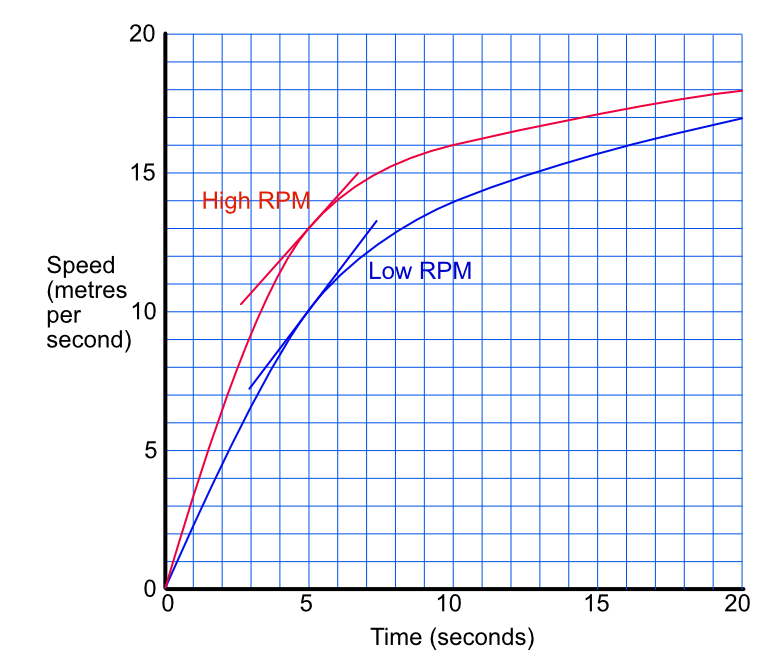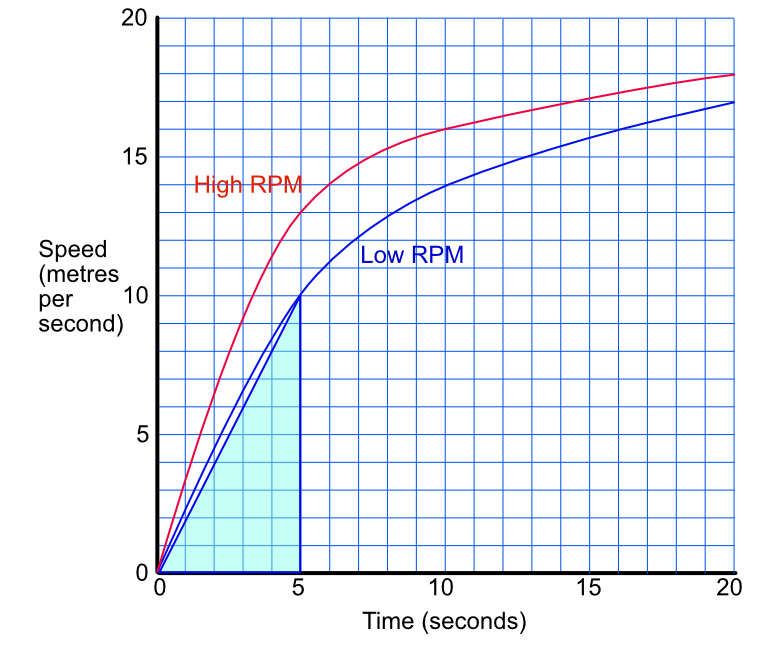Interpret Gradients and Areas in Context

# Interpret Gradients and Areas in Context

GCSE(H),

A gradient for a graph gives the rate of change of the item being measured. The area under a graph gives the amount consumed over an interval.

If the measurement is distance, then the rate of change of distance is velocity. If the measurement is velocity, then the rate of change gives acceleration. If the measurement is velocity, then the area gives the distance.

## Examples

1. The graph shows the performance of two similar engines. One runs at a higher rate of rpm (revolutions per minute) than the other. Which engine configuration has the higher rate of acceleration at 5 seconds?At 5 seconds, the gradient for High RPM is frac(3.850-2.650)(4) = 0.300 ms-2. For Low RPM the gradient is frac(3.200-1.800)(4) = 0.350ms-2.